Ex 4.3

Chapter 4 Class 10 Quadratic Equations (Term 2)
Serial order wise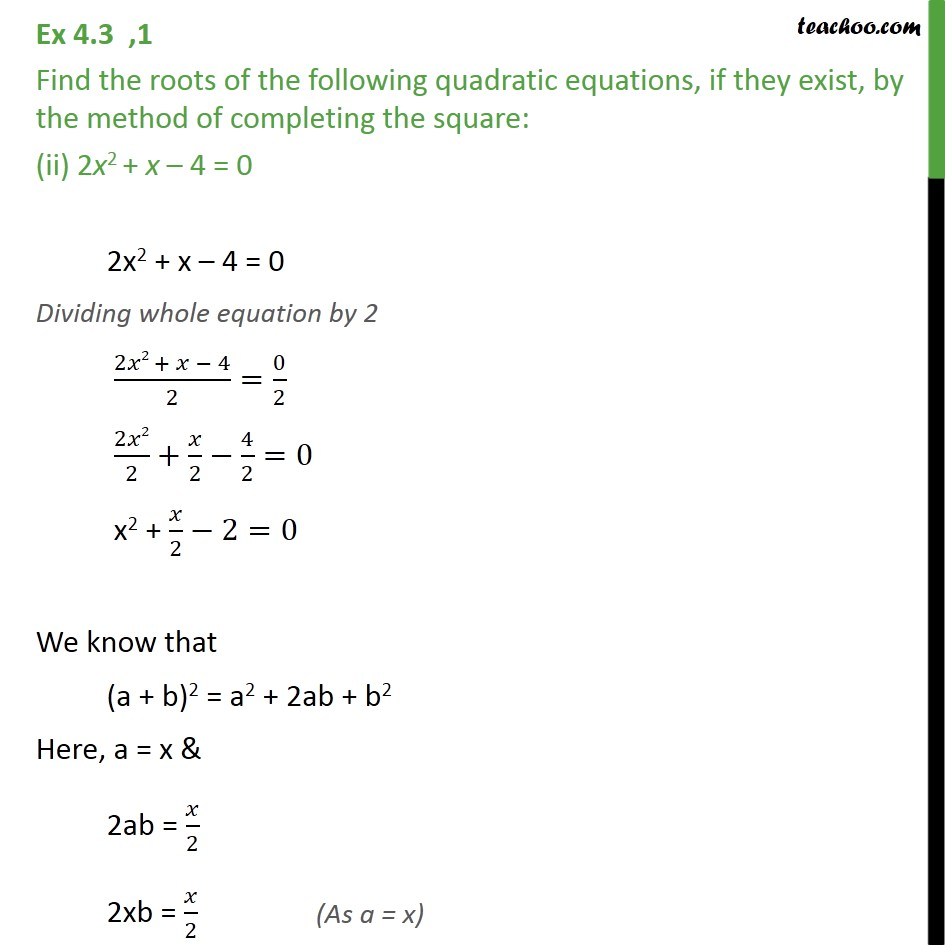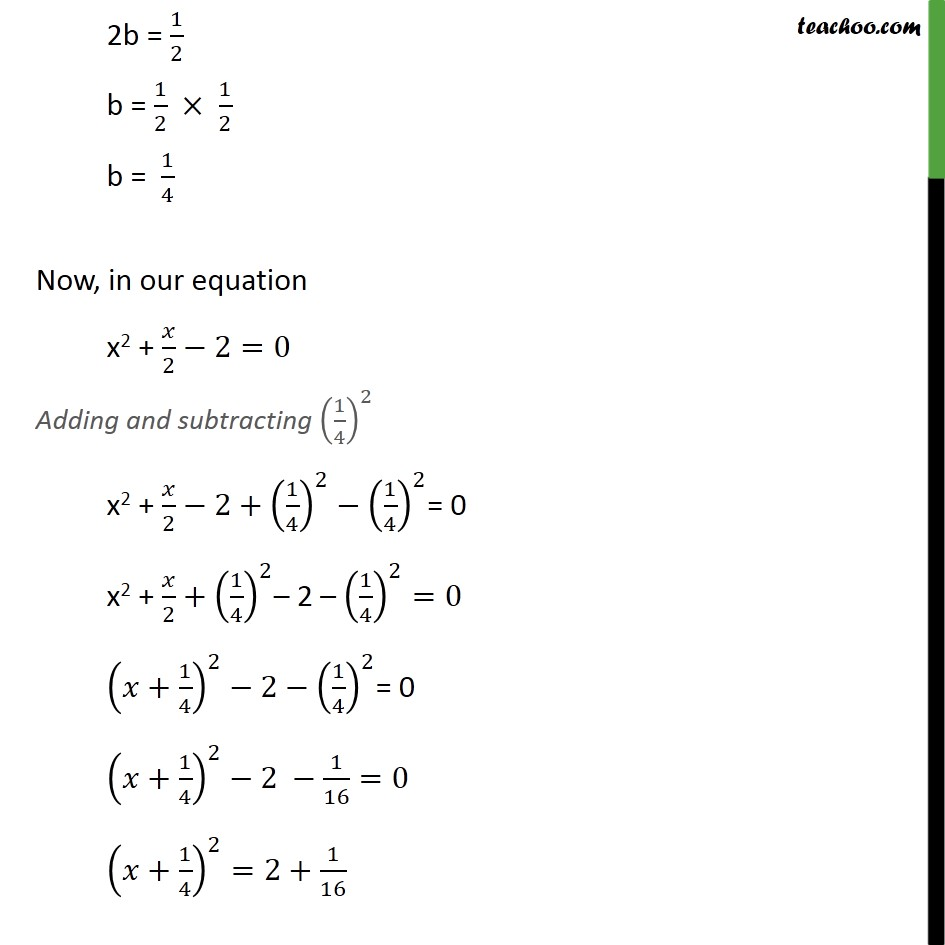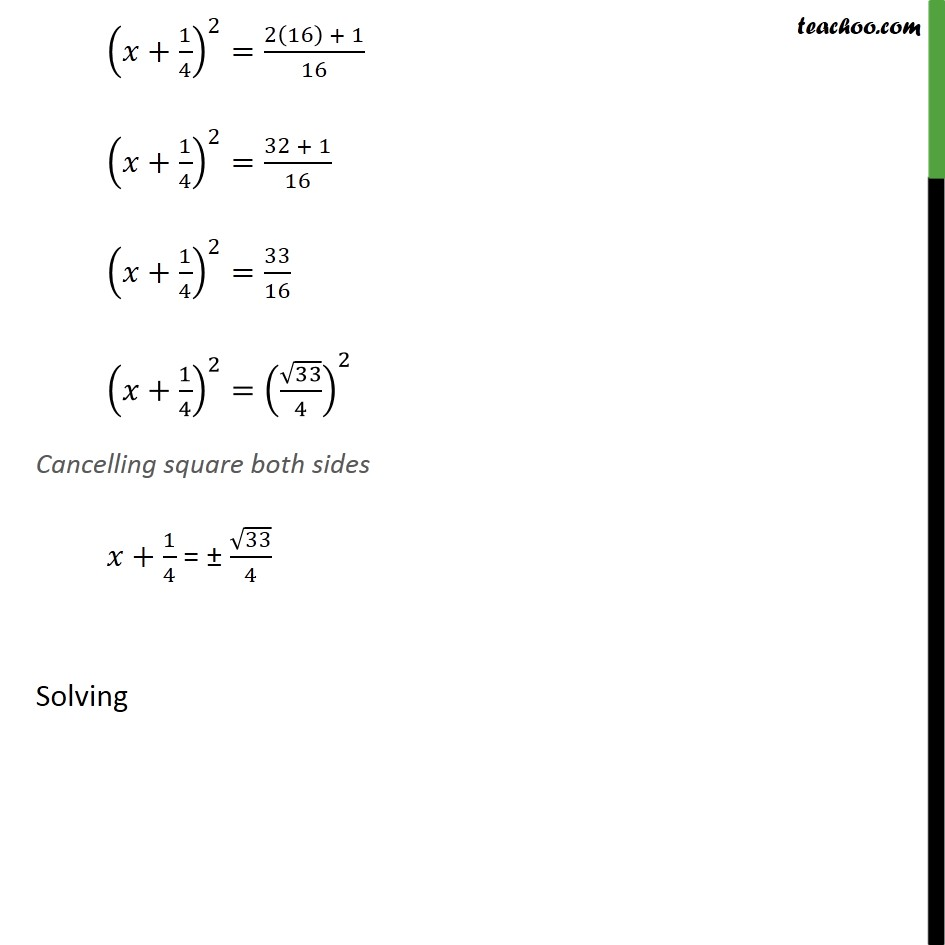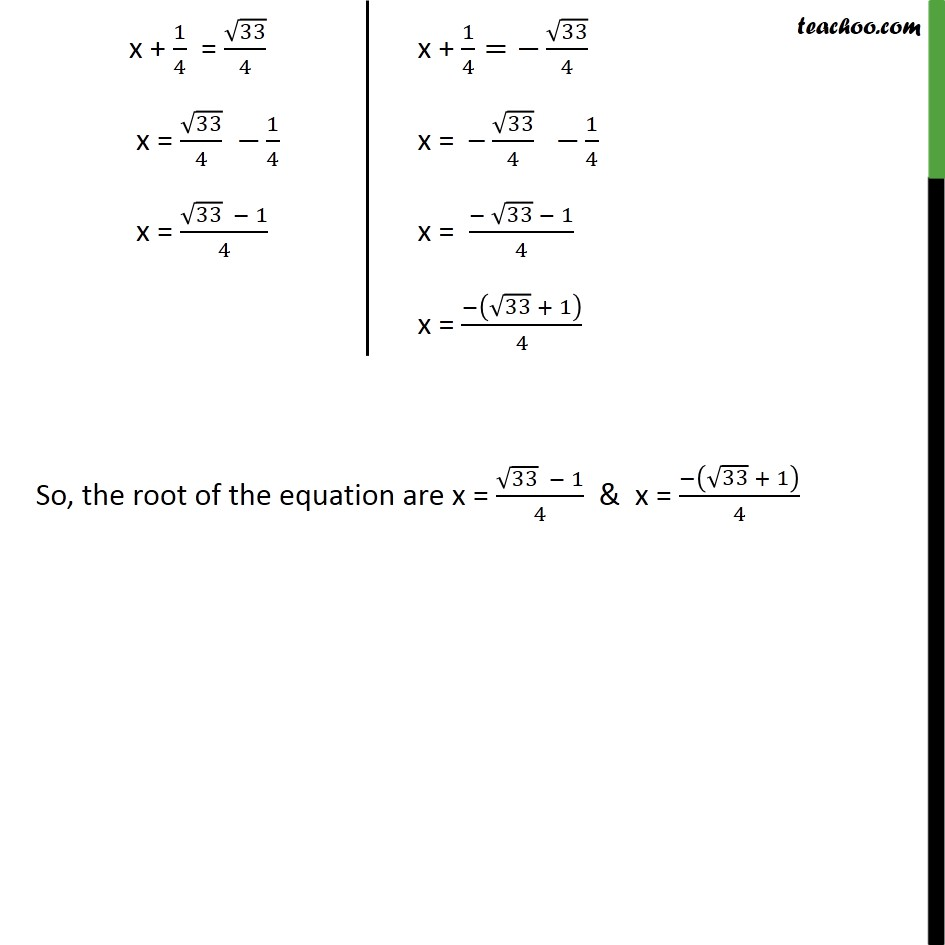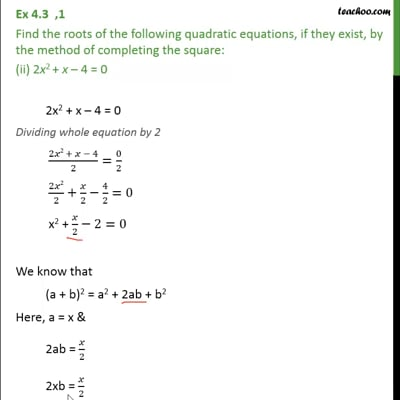This video is only available for Teachoo black users

### Transcript

Ex 4.3 ,1 Find the roots of the following quadratic equations, if they exist, by the method of completing the square: (ii) 2x2 + x – 4 = 0 2x2 + x – 4 = 0 Dividing whole equation by 2 (2𝑥2 + 𝑥 − 4)/2=0/2 2𝑥2/2+𝑥/2−4/2=0 x2 + 𝑥/2−2=0 We know that (a + b)2 = a2 + 2ab + b2 Here, a = x & 2ab = 𝑥/2 2xb = 𝑥/2 2b = 1/2 b = 1/2 × 1/2 b = 1/4 Now, in our equation x2 + 𝑥/2−2=0 Adding and subtracting (1/4)^2 x2 + 𝑥/2−2+(1/4)^2−(1/4)^2= 0 x2 + 𝑥/2+(1/4)^2– 2 – (1/4)^2=0 (𝑥+1/4)^2−2−(1/4)^2= 0 (𝑥+1/4)^2−2 −1/16=0 (𝑥+1/4)^2=2+1/16 (𝑥+1/4)^2=(2(16) + 1)/16 (𝑥+1/4)^2=(32 + 1)/16 (𝑥+1/4)^2=33/16 (𝑥+1/4)^2=(√33/4)^2 Cancelling square both sides 𝑥+1/4 = ± √33/4 Solving So, the root of the equation are x = (√33 − 1)/4 & x = (−(√33 + 1))/4